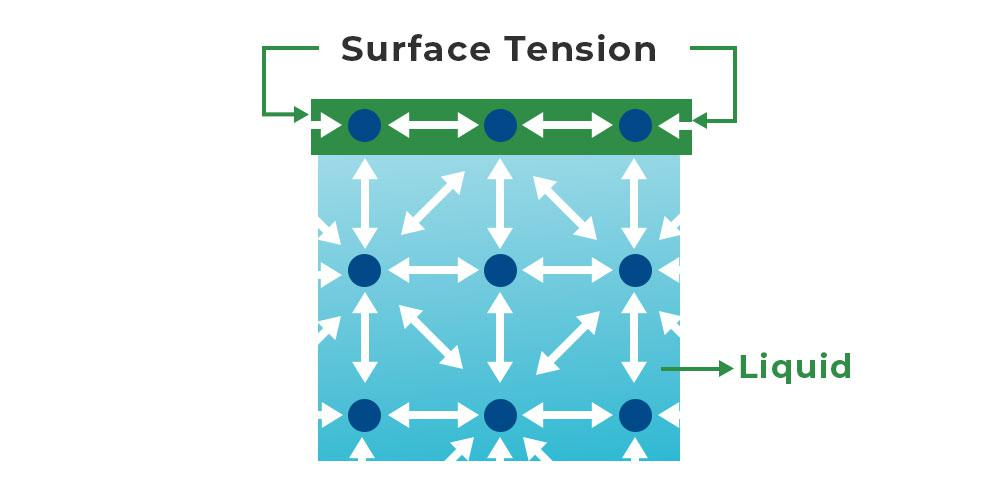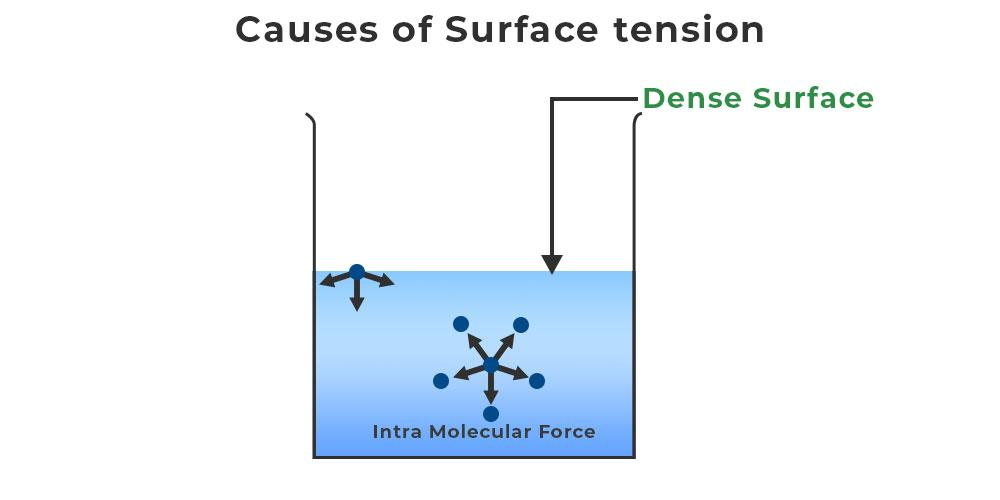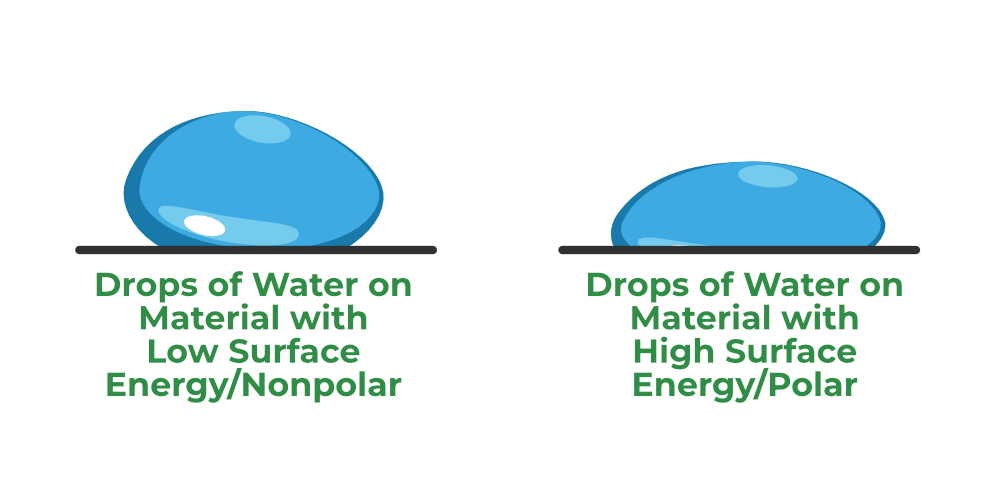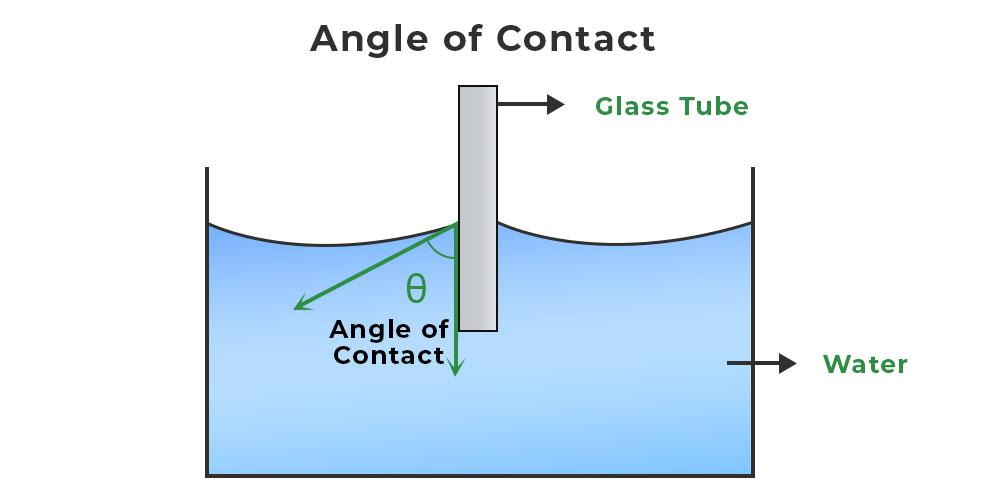GFG App
Open AppBrowser
Continue

Surface tension is the ability of fluid surfaces to contract into the smallest possible surface area. Have you ever found that even after filling a glass full of water, you can only add a few more drops before it spills? Have you ever lost a thermometer and watched how the mercury reacts as it falls? All of which are caused by the surface tension of the surface. Its isolated surface behaves like a strong rubber membrane due to the suppressive force in the fluid molecules. As a result, the individual surface of the fluid is still in a state of stress and tends to have the smallest field. Thus, Surface Tension refers to the tension on the fluid’s individual surface.

## Surface Tension Definition

Surface tension is described as the phenomenon that occurs when the surface of a liquid comes into contact with another phase (it can be a liquid as well). Liquids appear to have the smallest possible surface area. The liquid’s surface looks like an elastic sheet.

Imagine a line XY (as shown in the figure below) on the independent surface of the fluid in the equilibrium, then at every point on this line, the same force acts in its exact opposite direction. Every point stretched with the same force in both directions.Thus, in the equilibrium, a force acting on any other per unit length of an imaginary line on an independent surface of the fluid, which is perpendicular to the line and in the direction of the tangent line of the surface, is called Surface Tension.

Surface tension is denoted by σ or T symbol.

## Cohesion and Surface Tension

Surface Tension at liquid molecules is generated by the cohesion force among the atoms of the liquid. The cohesion force is the attractive force between two particles of Solid and Liquid. Cohension force is the force required to hold the solid and liquid particles together.

Surface Tension is the property of the substance because of the cohesion forces. The surface tension resists the change in the structure of the surface.

## Surface Tension at Molecular Level

Due to the Cohesion force the water molecule tends to stick together. The water molecule at the bottom layer has various molecules above them to stick but the molecule at the top layer does not have various other molecules to cling together. Thus, they attach to each other with a larger force and resist any change in their structure.

The molecule inside the body of the liquid experiences the forces from all directions and thus, the net force cancels out each other, whereas the particle at the top layer experiences a strong inward force resulting in the surface tension of the water. Because of this water has one of the highest surface tension among liquids.

## Formula for Surface Tension

Mathematically, the surface tension is defined as the force (F) acting on the surface and the length (l) of the surface, so is given as:

T = F / l

Also, the ratio of the work done (W) and the change in the area of the surface (A) is termed surface tension.

T = W / A

## Unit of Surface Tension

Surface tension is the ratio of the dragging force to the length and thus its SI unit is N/m as force is measured in N and length is measured in m. In the CGS system, its unit is Dyne/cm.

## Dimension of Surface Tension

The dimension formula of Force is [MLT-2] whereas the dimension of length is [L] thus, the dimensional formula of Surface Tension is [M L0 T-2].

## What is the Causes of Surface Tension?

The effect known as surface tension is caused by the cohesive forces between liquid molecules. Since the molecules at the surface lack like molecules in both directions, they cohere more closely to those specifically aligned with them on the surface. This creates a surface “film” that makes moving an object across the surface more difficult than moving it while fully submerged.Assume a jar is filled with water; the water molecules can be found in two positions in this jar: First, beneath the water, and second, on the surface of the water. Since there are no molecules above these molecules, the molecules at the water’s surface are unbalanced. As a result, only the molecules below will be attracted. As a result, a thin crust will form on the liquid’s upper surface. Because of this thick layer, a form of stress is generated, which is known as Surface Tension. These phenomena can also be explained in terms of energy.

## How to Calculate Surface Tension?

The surface Tension of any substance can easily be calculated using the surface tension formula as discussed above in the article. Let’s take an example to understand the calculation of Surface Tension in detail.

Example: Find the surface tension of the liquid with a dragging force of 12 N when the length at which the force acts is 4 m.

Solution:

Given,

F = 12 N
L = 4 m

According to the Surface Tension formula, T = F/L

T = 12/4

T = 3 N/m

## Methods of Measurement

Various methods which are used to measure the surface tension of any liquid are,

• Capillary Rise Method
• Bubble Pressure Method
• Spinning Drop Method
• Du Noüy Ring Method
• Stalagmometric Method
• Sessile Drop Method

Other than these there are various other methods that are used for measuring the surface tension of the liquids.

## What is Surface Energy?

Surface energy measures the breakdown of intermolecular bonds caused by the formation of a surface. Surface-free energy and interfacial-free energy are other names for it. Surface energy is defined as the work done per unit area by the force that forms the new surface.

The image given below shows the surface of the water molecules.When the free surface area of a liquid is increased, effort must be done against the force of surface tension. This work is stored as potential energy on the liquid surface. This increased potential energy per unit area of the free surface of the liquid is referred to as surface energy.

Mathematically, the surface energy is defined as:

Surface energy = Surface tension × Change in the surface area

or

ES = T × ΔA

where T denotes surface tension and ΔA denotes an increase in surface area.

Therefore, the SI unit of surface energy is Nm-2 and the dimensional formula is [MT– 2].

## What is Angle of Contact?

The angle of contact is defined as the angle subtended between the tangents drawn at the liquid surface and the solid surface within the liquid at the point of contact, or it is defined as the angle subtended between the tangents drawn at the liquid surface and the solid surface within the liquid at the angle of contact (θ).The angle of contact depends on the following factors:

• The nature of the liquid, the solid with which it comes into contact.
• The medium that exists above the free surface of the liquid.
• As the temperature of the liquid rises, so does the angle of contact.
• When soluble impurities are added to a liquid, the angle of contact drops.

## Examples of Surface Tension

Various examples which are explained with the help of Surface tension are,

### Walking on Water

Various insects can easily walk on the surface of the water because the force of their weight is not enough to penetrate the surface of the water.

### Floating Needle

A needle made of steel can easily be made to float on the surface of the water even though it is many times denser than water because of the surface tension of the water.

### Spherical Shape of Water Droplets

Small droplets of fluid are spherical due to surface tension. The molecules of water tend to stick together due to intramolecular force, and the energy of molecules that are located on the surface of droplets contains higher energy and try to push the other molecule to the centre of the droplet. Due to this the drop makes the shape that contains the least surface area and the spherical shape is best for the least surface area, that’s why The droplets of water and raindrops are spherical.

### Fire Polishing of Glass

The method of polishing glass or thermoplastic with the help of fire or flame is called Fire Polishing. When we heat a glass material in flames, the glass surface starts melting. But due to surface tension, it starts to become soft and smooth which makes the glass very flat and smooth. This method is most applicable to flat external surfaces. Flame polishing is frequently in acrylic plastic fabrication because of its high speed compared to abrasive methods. In this application, an oxyhydrogen torch is typically used, one reason being that the flame chemistry is unlikely to contaminate the plastic.

### Soaps and Detergents

Soaps and Detergents can easily clean clothes because they lower the surface tension of the water and thus allowing it to easily soak the grease and soil particles and then remove them.

### Rise of Liquids in Capillary Tubes

A tube whose radius is very short and uniform is called a capillary tube. When an open capillary is dipped in water. The water rises to some height in the capillary tube.

## Factors affecting Surface Tension

Various factors which affect the Surface Tension of any liquid are,

• If the solute is highly soluble in the fluid, the surface tension of the fluid would increase. And if the solute is less soluble in the fluid, then the surface tension of the fluid would decrease.
• If there are dust particles or any lubricant present on the surface of the fluid, the surface tension of the fluid decreases.
• Increasing the temperature reduces the surface tension of the fluid. And decreasing the temperature increases the surface tension.

## Solved Example on Surface Tension

Example 1: Find the surface tension of the liquid with a dragging force of 20 N when the length at which the force acts is 5 m.

Solution:

Given,

F = 20 N
L = 5 m

According to the Surface Tension formula, T = F/L

T = 20/5

T = 5 N/m

Thus, the surface tension of the liquid is 5 N/m

Example 2: Find the dragging force on the 2 m surface of the liquid if the surface tension is 10 N/m

Solution:

Given,

T = 10 N/m
L = 2 m

According to the Surface Tension formula, T = F/L

10 = F / 2

F = 10 × 2

F = 20 N

Thus, the dragging force is 20 N.

Example 3: Find the surface tension of the liquid with a dragging force of 26 N when the length at which the force acts is 2 m.

Solution:

Given,

F = 26 N
L = 2 m

According to the Surface Tension formula, T = F/L

T = 26/2

T = 13 N/m

Thus, the surface tension of the liquid is 13 N/m

Example 4: Find the dragging force on the 3 m surface of the liquid if the surface tension is 9 N/m

Solution:

Given,

T = 9 N/m
L = 3 m

According to the Surface Tension formula, T = F/L

9 = F / 3

F = 9 × 3

= 27 N

Thus, the dragging force is 27 N.

Related Articles

## FAQs on Surface Tension

### Question 1: What is Surface Tension?

The attraction caused by the particles of any liquid at their surface that tends to resist the change is called as Surface Tension. It is the force applied on the surface of liquid per unit of its length.

### Question 2: What is the Unit of Surface Tension?

The SI unit of Surface Tension is N/m whereas in CGS system it is measured in dyn/cm

### Question 3: When the temperature is increased what happens to the surface tension of the water?

The increase in the temperature decreases the surface tension of the water as increasing the temperature increases the kinetic energy of the molecules and thus the intermolecular attraction decreases.